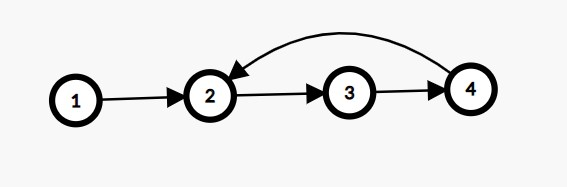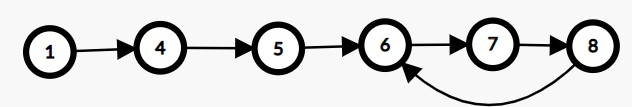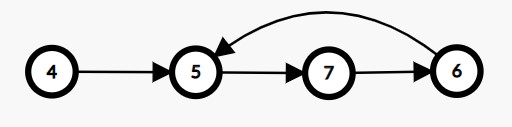New update is available. Click here to update.

# Remove Loop

Contributed by
Dipanshu Pandey
Last Updated: 23 Feb, 2023
Easy0/40Share2 upvotes

## Problem Statement

#### Refer example for better understanding.

##### Example :
`````` N = 4
K = 1
NODE = 1->2->3->4
````````````You can see there is a loop from the ‘1st’ node to the ‘3rd’ node (0 based), so we will remove all nodes from (1 - 3), so the answer is [ 1 ].
``````
Detailed explanation ( Input/output format, Notes, Images )##### Constraints :
``````1 <= T <= 10
1 <= N <= 1e5
0 <= K < N

Sum of N <= 10^5

Time Limit: 1 sec
``````
##### Sample Input 1 :
``````2
1 4 5 6 7 8 -1
3
4 5 7 6 -1
1
``````
##### Sample Output 1 :
``````1 4 5
4
``````
##### Explanation Of Sample Input 1 :
``````For test case 1,
Initially the racecourse looks like,
````````````After removing, 6->7->8 (Nodes in the loop), our final LinkedList looks like this.
````````````For test case 2,
Initially, the racecourse looks like,
````````````After removing, 6->7->8 (Nodes in the loop), our final LinkedList looks like this.
``````##### Sample Input 2 :
``````2
1 4 5 6 -1
3
1 3 -1
1
``````
##### Sample Output 2 :
``````1 4 5
1
``````AutoConsole